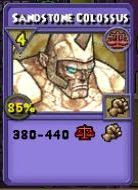## Wednesday, January 25, 2012

### New Level 70 Crowns Gear

Personally, I think only the Axe is worth the crowns... All the rest is meh. I totaled the gear stats and posted pictures of the new spells.

Level 70 Crowns Gear Key
H = Health
E = Energy
AP= Armor Piercing
_R= School Resist (G = General)
ST= Stun Resist
_D= School Damage (G = General)
_A= School Accuracy (G = General)
_B= School Block (G = General)
_C= School Critical (G = General)
IH= Incoming Heal
OH= Outgoing HealLevel 70 Wand - Crescent Axe of the Astrals - 5995C
AP = 3%
GB = 30
GC = 20
IH = 12%
+1 Power Pip
6 Cards: 0 Pip 130 Moon Damage

Level 70 Gear - 12500C / Set
Generic - Splendid Attire
H = 204 + 271 + 175 = 650
E = 15 + 15 + 15 = 45
AP= 1% + 1% + 1% = 3%
GB= 25 + 20 + 30 = 75
GR= 7% + 6% + 7% = 20%
ST= 15% + 10% + 20% = 45%
Cards: 2x Empower + 2x Sandstone Colossus (4 Pip, 380-440 Balance Damage), 1x Spirit Defuse + 1x Elemental DefuseMyth - Sly Rogue Attire
H = 274 + 400 + 120 = 794
AP= 2% + 3% + 3% = 8%
DR= 7% + 10% + 8% = 25%
FR= 5% + 8% + 6% = 17%
LR= 7% + 9% + 8% = 24%
MC= 65 + 35 + 50 = 150
MD= 9% + 15% + 11% = 35%
Cards: 2x Vaporize, 2x War Helephant (4 pip, 345-405 Myth Damage), 2x ShatterDeath - Forlorn Soul Attire
H = 312 + 492 + 216 = 1020
AP= 1% + 1% + 2% = 4%
DC= 70 + 35 + 45 = 150
DD= 9% + 12% + 11% = 32%
IR= 5% + 9% + 7% = 21%
LR= 9% + 13% + 12% = 34%
MR= 6% + 10% + 10% = 26%
Cards: 2x Dark Pact, 2x Death helephant (4 pip, 380-440 Death Damage), 2x StrangleBalance - Decorous Paragon Attire
H = 363 + 500 + 307 = 1170
AP= 1% + 1% + 2% = 4%
BC= 80 + 40 + 40 = 160
BD= 8% + 10% + 8% = 26%
FR= 10% + 9% + 9% = 28%
IR= 9% + 9% + 8% = 26%
SR= 11% + 10% + 10% = 31%
Cards: 2x Black Mantle, 2x Plague Ninja Pig, 2x Unbalance

Storm - Lightning Dancer Attire
H = 133 + 230 + 89 = 452
AP= 4% + 2% + 5% = 11%
BR= 6% + 6% + 5% = 17%
FR= 6% + 5% + 5% = 16%
LR= 5% + 3% + 3% = 11%
SC= 75 + 30 + 45 = 150
SD= 10% + 11% + 8% = 29%
Cards: 2x Dissipate, 2x Supercharge, 2x Storm Cat (0 Pip, 80-120 Storm Damage)Ice - Whiteblind Attire
H = 253 + 379 + 136 = 768
GB= 75 + 35 + 40 = 150
GR= 7% + 7% + 6% = 20%
ST= 5% + 5% + 5% = 15%
Cards: 2x Melt, 2x Ice Hound, 2x Legion Shield

Fire - Fire Dancer Attire
H = 278 + 422 + 146 = 846
AP= 3% + 3% + 2% = 8%
BR= 7% + 9% + 8% = 24%
FC= 70 + 45 + 40 = 155
FD= 10% + 11% + 10% = 31%
IR= 6% + 7% + 6% = 19%
SR= 8% + 9% + 9% = 26%
Cards: 2x Smoke Screen, 2x Quench, 2x Magma Colossus (4 Pip, 480 Fire Damage, -45% Shield to next Damage Spell)Life - Springstep Attire
H = 327 + 465 + 163 = 955
AP= 1% + 2% + 1% = 4%
DR= 8% + 9% + 9% = 26%
LC= 65 + 40 + 30 = 135
LD= 8% + 7% + 9% = 24%
MR= 7% + 9% + 8% = 24%
SR= 6% + 7% + 7% = 20%
Cards: 2x Entangle, 2x Brilliant Light, 2x Bronze Helephant